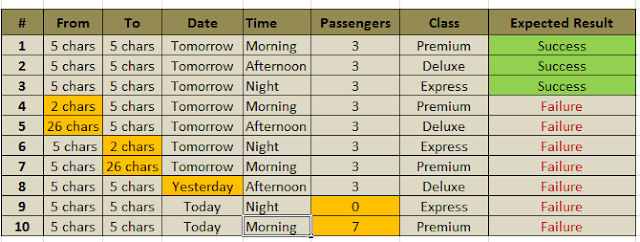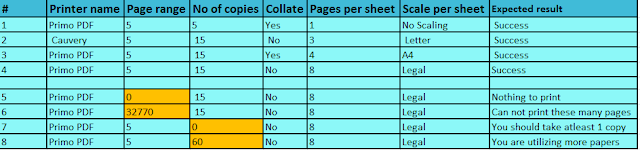## November 12, 2018

Question
One of your friends has written a program to search a string in a string and requested you to test the below function using equivalent class portioning :

function strpos_generic( \$haystack, \$needle, \$nth,  \$insensitive)
Following are terminology definitions:
• \$haystack= the string in which you need to search value
• \$needle= the character that needs to be searched, the \$needle should be a single character
• \$nth= occurrence you want to find, the value can be number between 1,2,3
• \$insensitive= 1- case insensitive, 0 or any other value is case sensitive
• Passing as Null as parameter in haystack or needle is not a valid scenario and will return boolean false
• The function will return mixed integer either the position of the \$nth occurrence of \$needle in \$haystack, or Boolean false if it can't be found.
a) Derive positive and negative domain equivalence class portioning with the value in the following format

 \$haystack \$needle \$nth \$insensitive

b) And drive test case for weak robust variant in following format and use the variable efficiently and cover  all the paths:

 Sl.no \$haystack \$needle \$nth \$insensitive Expected  results

Answer:
NOTE : The above question is for your exercise,kindly try solving it using below reference questions

Question
ABC Transportation Company has implemented an online ticket booking system for their buses, and they have created a simple screen to search the bus and following are some of the business rules they have incorporated in the screen.
From and To location should be minimum 3 characters and maximum  of  25 characters
Date of travel cannot be lesser than today’s date
Time : Morning, Afternoon, Night
No of Passengers can be 1 to 6
Service class can be: Premium, Deluxe, Express
a) Identify the positive and negative domain.
b) Write test case for weak robust variant.

Answer a)
Positive Domain

Negative DomainAnswer b)
Test case for weak robust variant : 3+7=10Question
A screen prototype for a expense management  system is given:

Following are the key business rules
• The date should be with in the same month and date should not be future date
• Negative values and zero amount is not accepted
• Claim amount should be less than Rs 10,000
• Payment method is a list box with “Cash, Debit card and credit card option” by default cash is selected
• Category of expense is a list box with “Travel, Food and Hotel” by default travel is selected
• On click of Save if all the business conditions are not meet then system will show an error “please fill right information”
A)Identify positive and negative domains in following format
B)Identify number of tests for Weak robust variants of equivalence class partitioning
C)Write test case with expected results in following format for weak robust variant

Answer A)
Positive

Negative
Answer B)
The number of tests for weak robust equivalence : 3+4=7
Answer C)
Test Cases

Question
The screen shot given is a customized print option from windows.
Following are the business rules available while printing.All drop down are non editable fields.
Name:
You can select “Primo PDF” or Network printer “Kaveri” from the name of the printer
Pages:
You can print page from 1 to 32767 pages. Given a number those many pages from first page will be printed. For example if we give pages=5 then first 5 pages will be printed. Any value that is given bellow 1 will get an error message “there is nothing to print”. Any value >32767 then you will get a message “cannot print these many pages”. If you print more than 20 pages a warning message “you are trying to print lot of pages” will be displayed. When you say All, All the active pages will be printed.
Number of copies:
You can take 1 to 50 copies. Any value that is given bellow 1 will get an error message “You should take atleast 1 copy”. Any value >50 then you will get a message “you are utilizing more papers”. If you print more than 10 copies a warning message “you are trying to take lot of copies” will be displayed.

a)Identify the Valid and Invalid sub domains using following table format:
Answer a)
Postive
Negative

b)Calculate the total number of test cases with formula for following different variants of equivalence class :
Weak normal ,Weak robust , Strong normal ,Strong robust

Answer b)
No of Test case:
• Weak normal : 4
• Weak robust: 8
• Strong normal : 128
• Strong robust : 768

c)Also derive the test cases for Weak robust variant in following format:

Answer c)
Test CasesQuestion
BITS-WASE has developed a new system to decide whether the student can be promoted to the next semester, Following are the rules
• Student should have got >= 50 and <=100 marks in the exam
• Student should have got >=5 and <=10 marks in the assignment
• Student should have >100 hrs. and <=128 hrs of class attendance
a) Identify number of test cases  for the following  :
• BVA
• Robust BVA
• Worst case BVA
• Robust worst case BVA
Answer)
Boundaries
Test Cases

• Basic BV= 4n+1=4*3+1=13
• Robust BV= 6n+1 = 6*3+1=19
• Worst case BV=5n=53=5*5*5=125
• Robust worst case BV =7n =73=7*7*7=343
Question
Construct decision table for the following specification of the insurance policy example:
When a new insurance policy is issued based on person’s Date of Birth, Policy anniversary date, premium Rate and number of claims(Initialized at zero) whenever  a claim message is sent ,the Number of is incremented.
When annual renewal message is called it evaluates the insured person’s age and number of claims to determine if the claim should be cancelled or if warning letter should be generated. It also determines a dollar amount representing an increase to the premium.
On the Following Rules:

1. If the person age is 25 or younger and they have 0 claims they will receive a \$50 Dollar increase in their insurance premium.
2. If the person is 26 or older and they have 0 claims they will receive \$25 dollar increase in their insurance premium.
3. If the person has 1 claim and they are :
•  26 or older – they get \$50 dollar increase
•  25 or younger – they  get a \$100 dollar increase and receive a warning letter.
4. If the person has 2 to 5 claims and they are :
•  26 or older – they get \$200 dollar increase
•  25 or younger – they  get a \$400 dollar increase and receive a warning letter.
5. if the person has 5+ claims their policy is canceled . No increase in premium

Answer

Question
For the following code.

1.  IF A = 5
2.  THEN IF B > C
3.        THEN A = B
4.    ELSE A= C
5.    ENDIF
6. ENDIF
7. Print A

a) Construct Control Flow Graph
b) Statement coverage path and Node coverage path of the CFG

Answer
a)Control Flow Graph
Cyclomatic complexity = E - N + 2
where,
E = number of edges in the flow graph.
N = number of nodes in the flow graph.

- The complexity of the program can be defined by three methods -
1. V(G) = E - N + 2 (where, E - Number of edge, N - Number of Nodes)
2. V (G) = P + 1 (where P = Number of predicate nodes (node that contains condition))
3. V(G)= number of Regions

The Cyclomatic complexity is calculated using the above control flow diagram that shows seven nodes(shapes) and eight edges (lines), hence the cyclomatic complexity is 8 - 7 + 2 = 3

b)
Statement coverage path :
• To calculate Statement  Coverage, find out the shortest number of paths following which all the nodes will be covered.
• So we can possibly traverse through the nodes in 2 ways.
• Therefore Statement Coverage is 2
Branch Coverage
• To calculate Branch  Coverage, find out the minimum number of paths which will
• ensure covering of all the edges. In this case there is no single path which will ensure coverage
• of  all the edges  at one go.
•  It covers both the true and false conditions unlike statement coverage.
• Therefore branch coverage is 3
Question
Find the Cyclomatic complexity and basis paths of the given CFG
a) Cyclomatic Complexity
Answer
Cyclomatic complexity = E - N + 2
Edges = 11
Nodes = 9

Therefore C = 11-9+2 = 4

b) Basis Paths
Answer:
Basis Set/Paths- A set of possible execution path of a program.
1,11
1,[2,3],[4,5],10,1,11
1,[2,3],6,7,9,10,1,11
1,[2,3],6,8,9,10,1,11

Similar questions for finding Cyclomatic Complexity,Basis Paths ,Statement Coverage and Branch Coverage

Question
Find Cyclomatic Complexity and basis paths for below CFG

Answer
Cyclomatic Complexity by 3 methods:
1. V(G) = 9 - 7 + 2 = 4
2. V(G) = 3 + 1 = 4 (Condition nodes are 1,2 and 3 nodes)
3. V(G)= Regions which are 4

Basis Set
1, 7
1, 2, 6, 1, 7
1, 2, 3, 4, 5, 2, 6, 1, 7
1, 2, 3, 5, 2, 6, 1, 7

Question
Find statement coverage path and Branch coverage path and Cyclomatic Complexity for below CFG:

Answer
Cyclomatic Complexity : E-N+2
Edges = 16
Nodes =13
Therefore C = 16-13+2=5
Statement Coverage :
• SCPath1: 1-2-3(F)-10(F)-11-13
• SCPath2: 1-2-3(T)-4(T)-5-6(T)-7(T)-8-9-3(F)-10(T)-12-13
Branch or Decision Coverage :
• BCPath1: 1-2-3(F)-10(F)-11-13
• BCPath2: 1-2-3(T)-4(T)-5-6(T)-7(T)-8-9-3(F)-10(T)-12-13
• BCPath3: 1-2-3(T)-4(F)-10(F)-11-13
• BCPath4: 1-2-3(T)-4(T)-5-6(F)-9-3(F)-10(F)-11-13
• BCPath5: 1-2-3(T)-4(T)-5-6(T)-7(F)-9-3(F)-10(F)-11-13
where T-True condition and F-False condition

Question
What is the significance of the following in Software Testing?

1.Permutation and Combination
Answer
A set of good test scenarios evaluates every possible permutation and combination of a program during ideal conditions.

2.Graph Theory
Answer
A graph is a pictorial representation of a set of objects where some pairs of objects are connected by links.
The interconnected objects are represented by points termed as vertices, and the links that connect the vertices are called edges
Degree of a node: the number of edges that have that node as an endpoint.

Question
What is Model Based testing? How Finite State Machine will help in Model based testing?

Answer
• A finite state machine is a directed graph in which states are nodes and transitions are edges.
• Source and sink states become initial and terminal nodes, paths are modeled as paths, and so on.
• Most finite state machine notations add information to the edges (transition) to indicate the cause of the transition called event and actions that occur as a result of the transition.
• Finite state machines can be executed, but a few conventions are needed first.
• One is the notion of the active state. The active state refers to the state “we are in”.
• Another convention is that FSM may have an initial state, which is the state that is active when a FSM is first entered.
• Exactly one state can be active at any time.
• To execute a FSM, we start with an initial state and provide a sequence of events that causes state transition.
• As each event occurs, the transition changes the active state and a new event occurs.
• In this way, sequence of evens selects a path of state (or equivalently of transitions) through the machine.